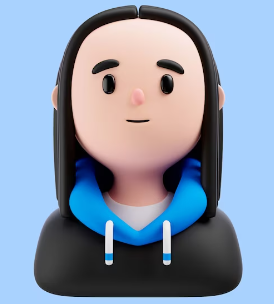#####GET QUOTE IN 2 MINUTES*(5/5)

### For small input instances, n 30, you may output your results to the command window. Larger input instances must be written to a file. In either case, you may need to produce an output file for trace runs or asymptotic performance analysis.

INSTRUCTIONS TO CANDIDATES

For small input instances, n 30, you may output your results to the command window. Larger input instances must be written to a file. In either case, you may need to produce an output file for trace runs or asymptotic performance analysis.

1. Closest Pairs [30 points]

There are several practical applications of this algorithm, such as, moving objects in a game, classification algorithms, computational biology, computational finance, genetic algorithms, and N -body simulations.

• [5 points] Construct a brute-force algorithm for finding the closest pair of points in a set of n points in a two-dimensional plane and show the worst-case big-O estimate for the number of operations used by the algorithm. Hint: First determine the distance between every pair of points and then find the points with the closest

• [5 points] Implement the brute-force algorithm from part (a).

• [10 points] Given a set of n points (x1, y1), ..., (xn, yn) in a two dimensional plan, where the distance be- tween two points (xi, yi) and (xj, yj) is measured by using the Euclidean distance (xi xj)2  + (yi      yj)2, design an algorithm to find the closest pair of points that can be found in an efficient way and the worst- case big-O

• [10 points] Implement the algorithm from part (c) with the following methods:
1. [5 points] The distance of the closest pair of points. Return the distance between closest single pair
• [5 points] The distance of the closest m pairs of points, where m (n-1). Return the distances between the closest m pairs of points.
1. Deterministic Turing Machine (DTM) [70 points/10 available extra credit points]

In this problem you will implement four variants of a DTM. A DTM consists of the following:

• A finite state control,
• A infinitely long tape divided into tape squares, and
• [30 points] Design and code software implementing a generic DTM. Your generic DTM should have a finite state control mechanism that can be tailored for each parts (b) - (e). Your implementation must address each of the DTM components: a finite state control, an infinitely long tape divided into tape squares, and a read-write
• [10 points] Tailor your DTM from (a) to implement
1. [5 points] Using the input  tape contents produce trace runs for your
2. [5 points] Define at least four other input tapes and produce trace runs for your
• [15 points] Tailor your DTM from (a) to implement binary addition - The tape will need to handle variable length binary inputs, g., tape values of 101100101 add 101 or add 101101101.

 Operation x + xt Result Carry 0 + 0 0 0 0 + 1 1 0 1 + 0 1 0 1 + 1 0 1

• [15 points] Tailor your DTM from (a) to implement binary subtraction - The tape will need to handle variable length binary inputs, g., tape values of 101100101 subtract 101 or subtract 101101.

Table 3: Binary Subtraction Rules

 Operation x − xt Result Borrow 0 − 0 0 0 0 − 1 1 1 1 − 0 1 0 1 − 1 0 0

• Extra Credit [10 points] Tailor your DTM from (a) to implement binary multiplication - The tape will need to handle variable length binary inputs, g., tape values of 101100101 multiply 101 or multiply 1011.

Table 4: Binary Multiplication Rules

 Operation x × xt Result 0 × 0 0 0 × 1 0 1 × 0 0 1 × 1 1

(5/5)

## Related Questions

##### . Introgramming & Unix Fall 2018, CRN 44882, Oakland University Homework Assignment 6 - Using Arrays and Functions in C

DescriptionIn this final assignment, the students will demonstrate their ability to apply two ma

##### . The standard path finding involves finding the (shortest) path from an origin to a destination, typically on a map. This is an

Path finding involves finding a path from A to B. Typically we want the path to have certain properties,such as being the shortest or to avoid going t

##### . Develop a program to emulate a purchase transaction at a retail store. This program will have two classes, a LineItem class and a Transaction class. The LineItem class will represent an individual

Develop a program to emulate a purchase transaction at a retail store. Thisprogram will have two classes, a LineItem class and a Transaction class. Th

##### . SeaPort Project series For this set of projects for the course, we wish to simulate some of the aspects of a number of Sea Ports. Here are the classes and their instance variables we wish to define:

1 Project 1 Introduction - the SeaPort Project series For this set of projects for the course, we wish to simulate some of the aspects of a number of

##### . Project 2 Introduction - the SeaPort Project series For this set of projects for the course, we wish to simulate some of the aspects of a number of Sea Ports. Here are the classes and their instance variables we wish to define:

1 Project 2 Introduction - the SeaPort Project series For this set of projects for the course, we wish to simulate some of the aspects of a number of

### Ask This Question To Be Solved By Our ExpertsGet A+ Grade Solution Guaranteed##### Lovedeep SinghComputer science
(/5)

Hire Me##### Onkar LapateComputer science
(/5)

Hire Me